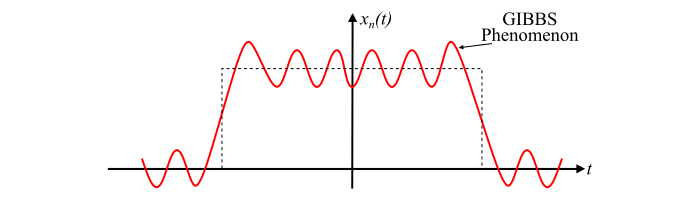# GIBBS Phenomenon for Fourier Series

## What is GIBBS Phenomenon?

The GIBBS phenomenon was discovered by Henry Wilbraham in 1848 and then rediscovered by J. Willard Gibbs in 1899.

For a periodic signal with discontinuities, if the signal is reconstructed by adding the Fourier series, then overshoots appear around the edges. These overshoots decay outwards in a damped oscillatory manner away from the edges. This is known as GIBBS phenomenon and is shown in the figure below.The amount of the overshoots at the discontinuities is proportional to the height of discontinuity and according to Gibbs, it is found to be around 9% of the height of discontinuity irrespective of the number of terms in the Fourier series. The exact proportion is given by the Wilbraham-Gibbs Constant.

$$\mathrm{\frac{1}{\pi}\int_{0}^{\pi}\frac{sin\:t}{t}dt-\frac{1}{2}= 0.089489 …}$$

It may also be noted that as more number of terms in the series are added, the frequency increases and the overshoots become sharper, but the amplitude of the adjoining oscillation reduces, i.e., the error between the original signal $x(t)$ and the truncated signal $x_{n} (t)$ reduces except at edges as the n increases. Hence, the truncated Fourier series approaches the original signal $x(t)$ as the number of terms in approximation increases.

## Effects of GIBBS Phenomenon

Following are some consequences of the GIBBS phenomenon −

• In signal processing, the GIBBS phenomenon is undesirable since it causes clipping from the overshoots and ringing artifacts from the oscillations.

• In MRI, the GIBBS phenomenon causes artifacts in the presence of adjacent regions of significantly differing signal intensity.

• The GIBBS phenomenon demonstrates a cross-pattern artifact in the discrete Fourier transform of an image, where the images have a sharper discontinuity between boundaries at the top-bottom and left-right of the image.